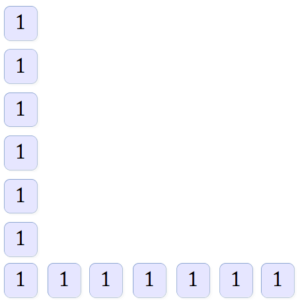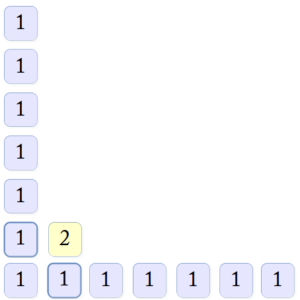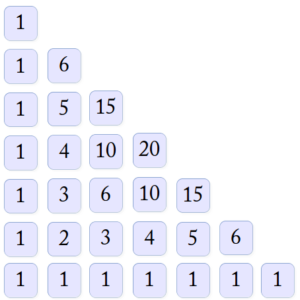# Metrics and Combinatorics: The Mēruprastāraḥ

We’ve seen one example of “spreading out” metrical patterns according to a certain rule in order to determine the number of possible patterns that can be made with k syllablic positions. There is a different procedure for determining the number of patterns, among the total, that contain a given number of light or heavy syllables. This procedure was called sarvaikādigalakriyā “working out the light and heavy syllables by starting with the number one in all positions” by Hēmacandra and other Jain authors (and hence it is Hēmacandra’s version that I will discuss here, since I am relying on Ludwig Alsdorf’s 1933 article), but it was known to other authors by the much punchier name Mēruprastāraḥ, “spreading out Mount Meru.” This technique, by the way, is the same as Pascal’s triangle, although, as the Wikipedia page notes in detail, the name should not be taken to imply that Pascal was the first person to discover or use the technique.

The technique is this. If you are dealing with k syllables, write out “1” k+1 times in a line, horizontally and vertically. For example, if k = 6:Then, going from left to right and from bottom to top, write the sum of each square and the square that is to its top-left in the square that is immediately above it. [Note that you could do this step also to generate the vertical column, since adding zero to the value written in the leftmost square in each line — that is, 1 — yields one.] For example:You follow this procedure for each square in the line, except for the last. Then you move up a line. You should end up with the following picture:This is Pascal’s triangle. It gives us a couple of interesting facts about the patterns of each class:

• Since k = 6, we can add all of the numbers on the hypotenuse of the triangle, and we will get the number of different syllabic patterns of a six-syllabled meter: 1 + 6 + 15 + 20 + 15 + 6 + 1 = 64.
• But the same triangle will give us the solutions for any value of k. So if we are interested in the number of patterns for k = 4, we can add 1 + 4 + 6 + 4 + 1 = 16 (as already shown here).
• The number of squares in the hypotenuse represents the number of combinations of different quantities of light and heavy syllables. This number will always be k + 1. Hence if k is 6, then there are 7 possibilities: 6 light syllables [and 0 heavy], 5 light [and 1 heavy], 4 light [and 2 heavy], 3 light [and 3 heavy], 2 light [and 4 heavy], 1 light [and 5 heavy], and 0 light [and 6 heavy].
• The number in the square in the series along the hypotenuse represents the number of combinations of decreasing numbers of light syllables. Hence for k = 6, there is 1 combination of all light syllables, 6 combinations of 5 light syllables and 1 heavy syllable, and so on. You can confirm this by comparing the number of combinations of a decreasing number of light syllables for k = 4 with the prastāraḥ given here.